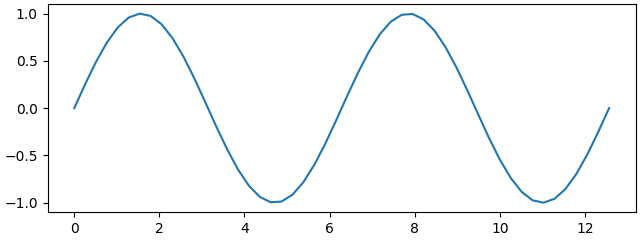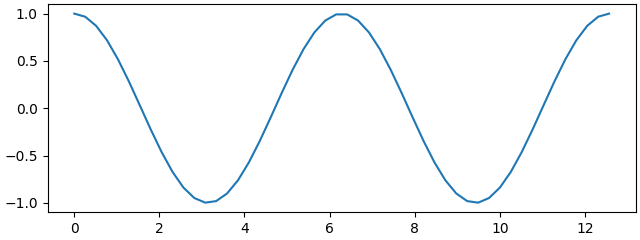# How to save subplots separately?

Hi, is it possible to save (to a png) all the subplots of a figure separately?

Yes, you can use `bbox_inches` keyword argument to `fig.savefig` . This takes a bounding box, in inches, and only saves that part of the rendered figure. The tricky part of this to get the right bounding box. If you want to slice your figure up by “figure fraction” (see Transformations Tutorial — Matplotlib 3.5.1 documentation to understand the coordinate systems in Matplotlib) you can do:

``````import matplotlib.pyplot as plt
import matplotlib.transforms as mtransforms

import numpy as np

th = np.linspace(0, 4 * np.pi)

fig, (ax1, ax2) = plt.subplots(2, constrained_layout=True)
ax1.plot(th, np.sin(th))
ax2.plot(th, np.cos(th))

fig.savefig(
"/tmp/bottom.png",
# we need a bounding box in inches
bbox_inches=mtransforms.Bbox(
# This is in "figure fraction" for the bottom half
# input in [[xmin, ymin], [xmax, ymax]]
[[0, 0], [1, 0.5]]
).transformed(
# this take data from figure fraction -> inches
#    transFigrue goes from figure fraction -> pixels
#    dpi_scale_trans goes from inches -> pixels
(fig.transFigure - fig.dpi_scale_trans)
),
)
fig.savefig(
"/tmp/top.png",
bbox_inches=mtransforms.Bbox([[0, 0.5], [1, 1]]).transformed(
fig.transFigure - fig.dpi_scale_trans
),
)
``````

The top:and the bottom:We do not have an automated way to extract just one sub axes from a figure. However, that might be a useful thing to put onto the new `SubFigure` objects?